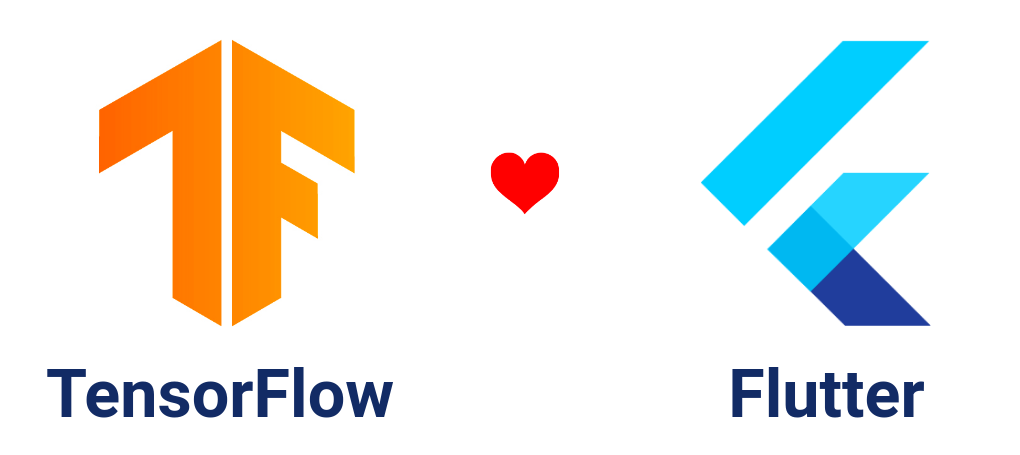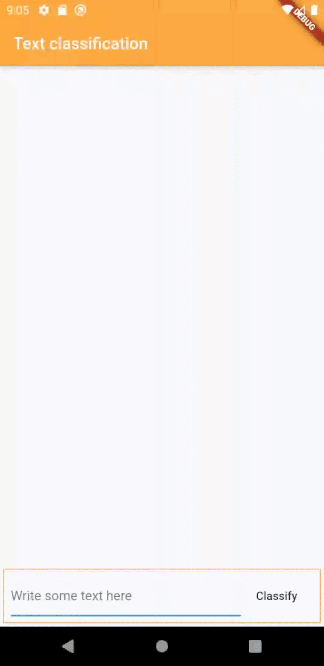# 在 Flutter 中使用 TensorFlow Lite 插件实现文字分类tflite_flutter 插件的核心特性：

• 它提供了与 TFLite Java 和 Swift API 相似的 Dart API，所以其灵活性和在这些平台上的效果是完全一样的
• 通过 dart:ffi 直接与 TensorFlow Lite C API 相绑定，所以它比其它平台集成方式更加高效。
• 无需编写特定平台的代码。
• 通过 NNAPI 提供加速支持，在 Android 上使用 GPU Delegate，在 iOS 上使用 Metal Delegate。

### （很重要）初始化配置

#### Linux 和 Mac 用户

`install.sh` 拷贝到您应用的根目录，然后在根目录执行 `sh install.sh`，本例中就是目录 `text_classification_app/`

#### Windows 用户

install.bat 文件拷贝到应用根目录，并在根目录运行批处理文件 `install.bat`，本例中就是目录 `text_classification_app/`

### 获取插件

`pubspec.yaml` 添加 `tflite_flutter: ^<latest_version>`详情）。

### 下载模型

`text_classification.tflite``text_classification_vocab.txt` 文件拷贝到 text_classification_app/assets/ 目录下。

`pubspec.yaml` 文件中添加 `assets/`

``````assets:
- assets/
``````

### 预处理

1. 对段落文本进行分词，然后使用预定义的词汇集将它转换为一组词汇 ID；
2. 将生成的这组词汇 ID 输入 TensorFlow Lite 模型里；
3. 从模型的输出里获取当前段落是积极或者是消极的概率值。

`lib/` 文件夹下创建一个新文件 `classifier.dart`

``````import 'package:flutter/services.dart';

class Classifier {
final _vocabFile = 'text_classification_vocab.txt';

Map<String, int> _dict;

Classifier() {
}

var dict = <String, int>{};
final vocabList = vocab.split('\n');
for (var i = 0; i < vocabList.length; i++) {
var entry = vocabList[i].trim().split(' ');
dict[entry] = int.parse(entry);
}
_dict = dict;
}

}
``````

``````import 'package:flutter/services.dart';

class Classifier {
final _vocabFile = 'text_classification_vocab.txt';

// 单句的最大长度
final int _sentenceLen = 256;

final String start = '<START>';
final String unk = '<UNKNOWN>';

Map<String, int> _dict;

List<List<double>> tokenizeInputText(String text) {

// 使用空格进行分词
final toks = text.split(' ');

var index = 0;
if (_dict.containsKey(start)) {
vec[index++] = _dict[start].toDouble();
}

// 对于句子里的每个单词在 dict 里找到相应的 index 值
for (var tok in toks) {
if (index > _sentenceLen) {
break;
}
vec[index++] = _dict.containsKey(tok)
? _dict[tok].toDouble()
: _dict[unk].toDouble();
}

// 按照我们的解释器输入 tensor 所需的形状 [1,256] 返回 List<List<double>>
return [vec];
}
}

``````

### 使用 tflite_flutter 进行分析

tflite_flutter 提供了一个方法直接通过资源创建解释器。

``````static Future<Interpreter> fromAsset(String assetName, {InterpreterOptions options})
``````

``````import 'package:flutter/services.dart';

// 引入 tflite_flutter
import 'package:tflite_flutter/tflite_flutter.dart';

class Classifier {
// 模型文件的名称
final _modelFile = 'text_classification.tflite';

// TensorFlow Lite 解释器对象
Interpreter _interpreter;

Classifier() {
// 当分类器初始化以后加载模型
}

// 使用 Interpreter.fromAsset 创建解释器
_interpreter = await Interpreter.fromAsset(_modelFile);
}

}

``````

``````void run(Object input, Object output);
``````

`Object input``Object output` 必须是和 Input Tensor 与 Output Tensor 维度相同的列表。

``````_interpreter.allocateTensors();
// 打印 input tensor 列表
print(_interpreter.getInputTensors());
// 打印 output tensor 列表
print(_interpreter.getOutputTensors());
``````

``````InputTensorList:
[Tensor{_tensor: Pointer<TfLiteTensor>: address=0xbffcf280, name: embedding_input, type: TfLiteType.float32, shape: [1, 256], data:  1024]
OutputTensorList:
[Tensor{_tensor: Pointer<TfLiteTensor>: address=0xbffcf140, name: dense_1/Softmax, type: TfLiteType.float32, shape: [1, 2], data:  8]
``````

``````int classify(String rawText) {

//  tokenizeInputText 返回形状为 [1, 256] 的 List<List<double>>
List<List<double>> input = tokenizeInputText(rawText);

// [1,2] 形状的输出
var output = List<double>(2).reshape([1, 2]);

// run 方法会运行分析并且存储输出的值
_interpreter.run(input, output);

var result = 0;
// 如果输出中第一个元素的值比第二个大，那么句子就是消极的

if ((output as double) > (output as double)) {
result = 0;
} else {
result = 1;
}
return result;
}

``````

``````// 将提供的列表进行矩阵变形，输入参数为元素总数 // 保持相等
// 用法：List(400).reshape([2,10,20])
// 返回  List<dynamic>

List reshape(List<int> shape)
// 返回列表的形状
List<int> get shape
// 返回列表任意形状的元素数量
int get computeNumElements

``````

``````import 'package:flutter/services.dart';

// 引入 tflite_flutter
import 'package:tflite_flutter/tflite_flutter.dart';

class Classifier {
// 模型文件的名称
final _modelFile = 'text_classification.tflite';
final _vocabFile = 'text_classification_vocab.txt';

// 语句的最大长度
final int _sentenceLen = 256;

final String start = '<START>';
final String unk = '<UNKNOWN>';

Map<String, int> _dict;

// TensorFlow Lite 解释器对象
Interpreter _interpreter;

Classifier() {
// 当分类器初始化的时候加载模型
}

// 使用 Intepreter.fromAsset 创建解析器
_interpreter = await Interpreter.fromAsset(_modelFile);
}

var dict = <String, int>{};
final vocabList = vocab.split('\n');
for (var i = 0; i < vocabList.length; i++) {
var entry = vocabList[i].trim().split(' ');
dict[entry] = int.parse(entry);
}
_dict = dict;
}

int classify(String rawText) {
// tokenizeInputText  返回形状为 [1, 256] 的 List<List<double>>
List<List<double>> input = tokenizeInputText(rawText);

//输出形状为 [1, 2] 的矩阵
var output = List<double>(2).reshape([1, 2]);

// run 方法会运行分析并且将结果存储在 output 中。
_interpreter.run(input, output);

var result = 0;
// 如果第一个元素的输出比第二个大，那么当前语句是消极的

if ((output as double) > (output as double)) {
result = 0;
} else {
result = 1;
}
return result;
}

List<List<double>> tokenizeInputText(String text) {
// 用空格分词
final toks = text.split(' ');

var index = 0;
if (_dict.containsKey(start)) {
vec[index++] = _dict[start].toDouble();
}

// 对于句子中的每个单词，在 dict 中找到相应的 index 值
for (var tok in toks) {
if (index > _sentenceLen) {
break;
}
vec[index++] = _dict.containsKey(tok)
? _dict[tok].toDouble()
: _dict[unk].toDouble();
}

// 按照我们的解释器输入 tensor 所需的形状 [1,256] 返回 List<List<double>>
return [vec];
}
}

``````

``````// 创建 Classifier 对象
Classifer _classifier = Classifier();
// 将目标语句作为参数，调用 classify 方法
_classifier.classify("I liked the movie");
// 返回 1 （积极的）
_classifier.classify("I didn't liked the movie");
// 返回 0 （消极的）
``````### 答疑

##### 问：`tflite_flutter` 和 `tflite v1.0.5` 有哪些区别？

`tflite v1.0.5` 侧重于为特定用途的应用场景提供高级特性，比如图片分类、物体检测等等。而新的 tflite_flutter 则提供了与 Java API 相同的特性和灵活性，而且可以用于任何 tflite 模型中，它还支持 delegate。

##### 问：如何使用 tflite_flutter 创建图片分类应用？有没有类似 TensorFlow Lite Android Support Library 的依赖包？

TensorFlow Lite Flutter Helper Library 为处理和控制输入及输出的 TFLite 模型提供了易用的架构。它的 API 设计和文档与 TensorFlow Lite Android Support Library 是一样的。更多信息请 参考这里